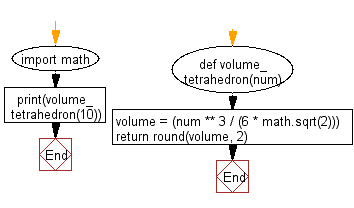﻿ Python Math: Calculate the volume of a tetrahedron - w3resource# Python Math: Calculate the volume of a tetrahedron

## Python Math: Exercise-64 with Solution

Write a Python program to calculate the volume of a tetrahedron.

Note: In geometry, a tetrahedron (plural: tetrahedra or tetrahedrons) is a polyhedron composed of four triangular faces, six straight edges, and four vertex corners. The tetrahedron is the simplest of all the ordinary convex polyhedra and the only one that has fewer than 5 faces.

Sample Solution:-

Python Code:

``````import math
def volume_tetrahedron(num):
volume = (num ** 3 / (6 * math.sqrt(2)))

return round(volume, 2)

print(volume_tetrahedron(10))
```
```

Sample Output:

```117.85
```

Pictorial Presentation:Flowchart:## Visualize Python code execution:

The following tool visualize what the computer is doing step-by-step as it executes the said program:

Python Code Editor:

Have another way to solve this solution? Contribute your code (and comments) through Disqus.

What is the difficulty level of this exercise?

Test your Programming skills with w3resource's quiz.

﻿

```>>> students = [{'name': 'John', 'score': 98}, {'name': 'Mike', 'score': 94}, {'name': 'Jennifer', 'score': 99}]